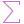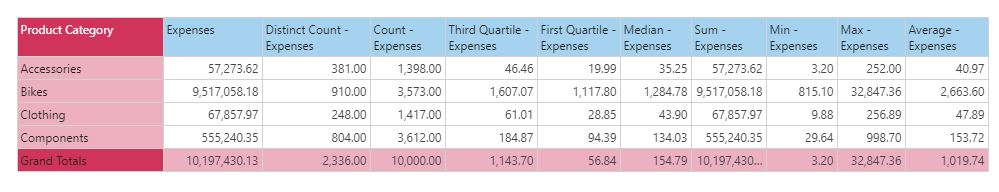#Count (PQL)

Executes count aggregation on a column of data from the model. The column does NOT need to have numerical data for it to be functional.

• Returned Output: Numeric
• Library: PQL \ Semantic \ Aggregate
• Version: 2018.00.000
• Compatibility: Pyramid Query Language (PQL) data sources

#### Syntax

Count( <Measure> )

* Click on the function's arguments above for more details on the input values.

• The "measure" column needs to be the name of the column in the model [table].[column name] or it can be an existing measure in the model [measures].[column name].
• For details on how to employ and use this function see the granular calculation overview.
##### Different Function types
• This function is like the SQL count function; calculating the count of items in a column of data in your query or model.
• If you wish to find the count for the elements in a specified SET or LIST from a hierarchy, use this semantic function. All counts are always distinct.
• To use a simple count mathematical operation on a list of numbers, use the Common library function.

#### Examples

To create a new measure that is the count of expenses in the model, using the sample demo model:

Count( [data].[Expenses] )

or :

Count( [measures].[data Expenses] )

The mechanics of this function are shown below. This grid says that the count expense across all 'Accessory' transactions is 1398. This means that counting the rows that are in the accessory category in the underlying table (transactions) is 1398. This produces a different result to the Count([LIST]) function which would simply count the number of elements in the list of items presented instead.